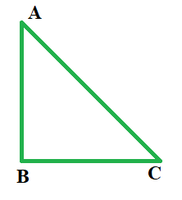Open in App
Not now

# Secant Formula

• Last Updated : 03 May, 2022

Trigonometry is a very important branch of mathematics which has a wide variety of applications. One such application pertains to the ratio of sides and angle measurements of triangles, particularly right-angled triangles.

In the figure below, we are given a right triangle ABC. Here, AC being the longest side, is the hypotenuse and AB is the perpendicular to the base BC.The different combinations of the ratios of these three sides of a given triangle yield the formulas for sin, cos, tan, cosec, sec and cot. Here we are going to discuss the secant formula.

Secant Formula

sec θ = Hypotenuse/Base

or,

sec θ = 1/cos θ

since cos θ = Base/Hypotenuse

Using the secant formula in the above figure, sec A would be AC/BC.

### Sample Problems

Problem 1. The value of cosine in a right triangle is 1/2. Find the value of the secant.

Solution:

The secant formula states that sec θ = 1/cos θ.

Since cos θ = 1/2

⇒ sec θ = 1/(1/2)

⇒ sec θ = 2

Problem 2. The hypotenuse and base of a right triangle are given to be 17 and 15 respectively. Find the value of the secant.

Solution:

sec θ = Hypotenuse/Base

Here, H = 17 and B = 15

⇒ sec θ = 17/15

Problem 3. Find the secant of a right triangle if its hypotenuse is 17 and perpendicular to the base is 8.

Solution:

Here, H = 17 and P = 8

As per Pythagoras Theorem, H2 = P2 + B2

B2 = 172 – 82

B = 15

Now, since sec θ = Hypotenuse/Base

⇒ sec θ = 17/15

Problem 4. The value of cosine in a right triangle is 0.1. Find the value of the secant.

Solution:

The secant formula states that sec θ = 1/cos θ.

Since cos θ = 0.1 = 1/10

⇒ sec θ = 1/(1/10)

⇒ sec θ = 10

Problem 5. The value of cosine in a right triangle is 0.2. Find the value of the secant.

Solution:

The secant formula states that sec θ = 1/cos θ.

Since cos θ = 0.2 = 1/5

⇒ sec θ = 1/(1/5)

⇒ sec θ = 5

My Personal Notes arrow_drop_up